# 实现

## A* 算法

A* 算法是一种启发式的寻路算法 ，对于任何一个点，它距离结束点的距离为 `f(n) = g(n) + h(n)`，其中 f 为总距离，g 为已确定的距离，h 为以某种方式估算到结束点的距离。

• 维护一个待访问的节点集合和一个已访问的节点集合；
• 每次从待访问的集合中取出 f 值最小的点 p ；
1. 检查是否已达到结算点

2. 获取点的邻居，标记当前点已访问并从待访问结合中删除

3. 依次访问每个邻居 n，如果邻居有效并且没有被标记已访问，计算 g 和 h. 其中 g 为点 p 的 g + 从 p 到 n 所需要的花费，h 一般采用曼哈段距离。

## 构建地图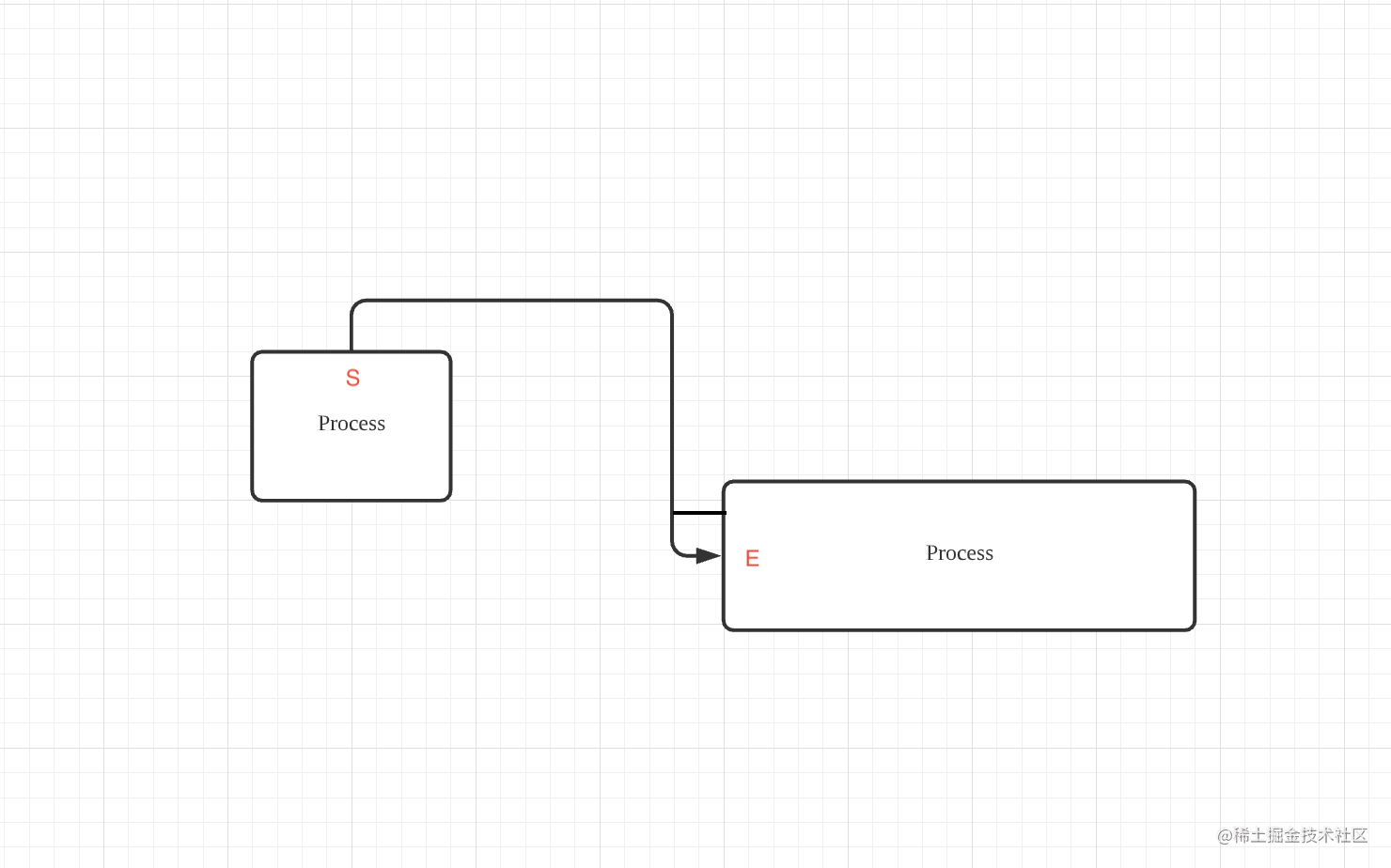`S``E` 的位置我们可以分为上下左右，定义如下：

``````enum Direction {
TOP = "top",
LEFT = "left",
RIGHT = "right",
BOTTOM = "bottom"
}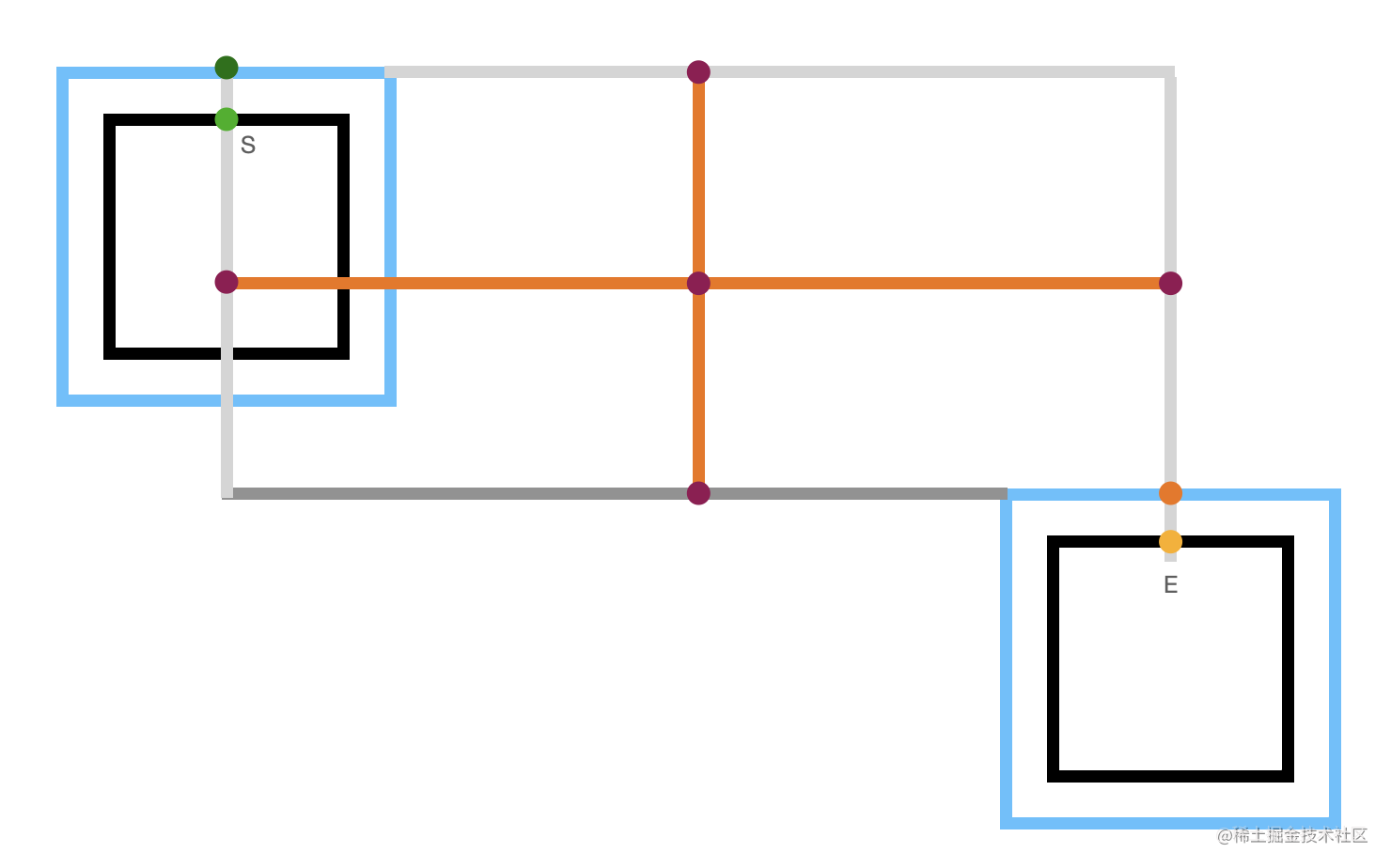## 路径查找

### 减少拐点

``````
const getDirection = (from: number[], to: number[]) => {
const v = subV(to, from);

if (v === 0) {
return v > 0 ? Direction.BOTTOM : Direction.TOP;
}

return v > 0 ? Direction.RIGHT : Direction.LEFT;
};

const lastDir = minP.parent ? getDirection(minP.parent.xy, xy) : null;
const turned = lastDir !== null && lastDir !== dir;
// 转向给些额外惩罚
const G = minP.G + grid.getCost(currentXY) + (turned ? 0.02 : 0);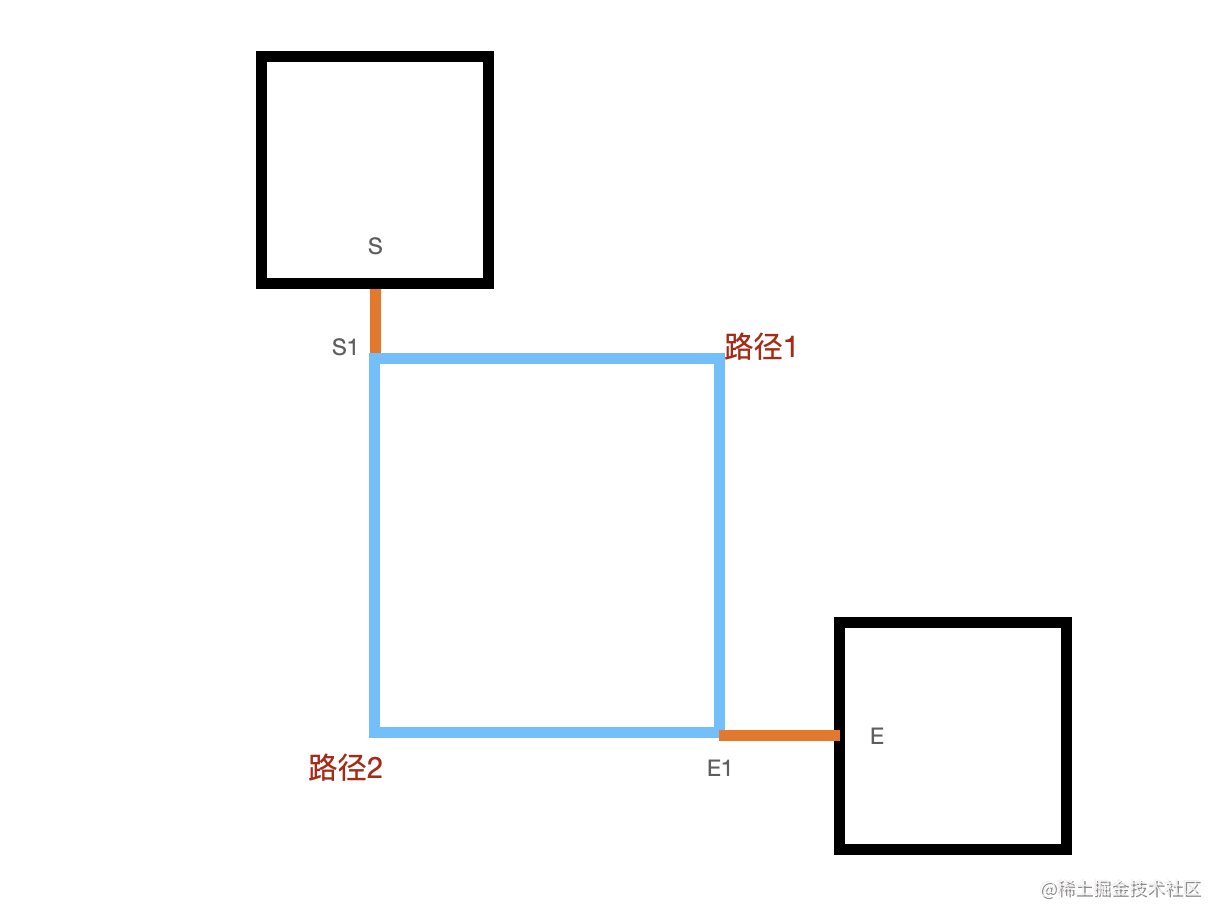``````    const move = getMoveDelta(startDirection, isFirst);
const neighbors =
index !== undefined
? isFirst
? move.slice(index, index + 1)
: move
: move;
isFirst = false;

`````` const result = [0, 1, 2, 4]
.map((index) =>
A(
grid,
startInfo.endpoint,
endInfo.endpoint,
startInfo.direction,
endInfo.direction,
heuristic,
index
)
)
.filter((item) => item.path.length);

let target: { path: number[][]; grid: Grid; G: number } | null = null;
let min1 = Infinity;
let min2 = Infinity;

result.forEach((item) => {
const completedPath = [...item.path];

completedPath.push(endInfo.origin);
completedPath.unshift(startInfo.origin);

const d1 = getNumberOfInflectionPoints(item.path);
const d2 = getNumberOfInflectionPoints(completedPath);

/**
* 1. 拐点数都相同时取最小的 G
* 2. 先取不包含起始点的最小拐点数，再判断包含了起始点的最小拐点数
*/
if (
d1 < min1 ||
(d1 === min1 && d2 < min2) ||
(d1 === min1 && d2 === min2 && item.G < target!.G)
) {
min1 = d1;
min2 = d2;
target = item;
}
});

### 合法的进入离开方向

``````const checkDirectionIsValid = (
from: number[],
to: number[],
direction: Direction
) => {
const d = subV(to, from);

let disabled = false;

switch (direction) {
case Direction.TOP:
disabled = d < 0;
break;
case Direction.LEFT:
disabled = d < 0;
break;
case Direction.RIGHT:
disabled = d > 0;

break;
default:
disabled = d > 0;
}

return !disabled;
};

// 查找到结束点时

if (minP.key === endP.key) {
if (
minP.parent &&
!checkDirectionIsValid(minP.parent.xy, xy, endDirection)
) {
continue;
}
...
break;
}

### 让路径经过某个点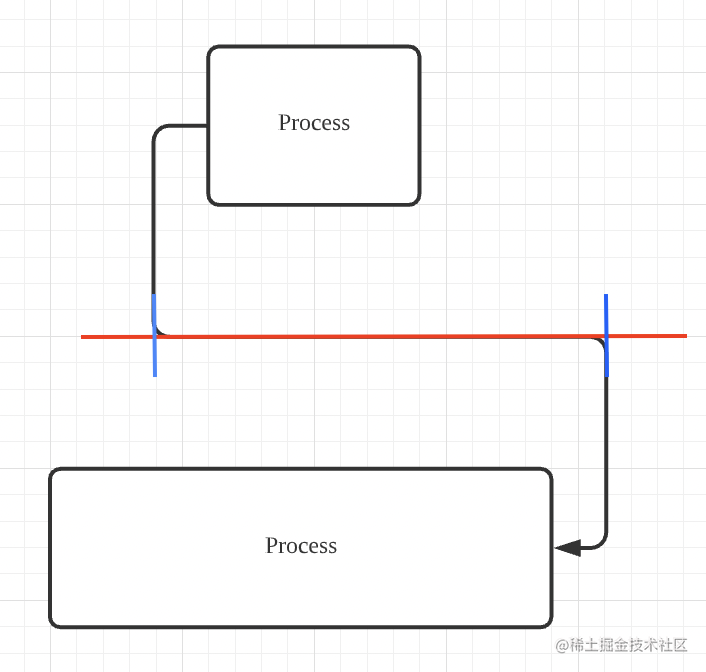### 对路径经过的区域作限制

#### 两个盒子足够远（蓝色矩形相交）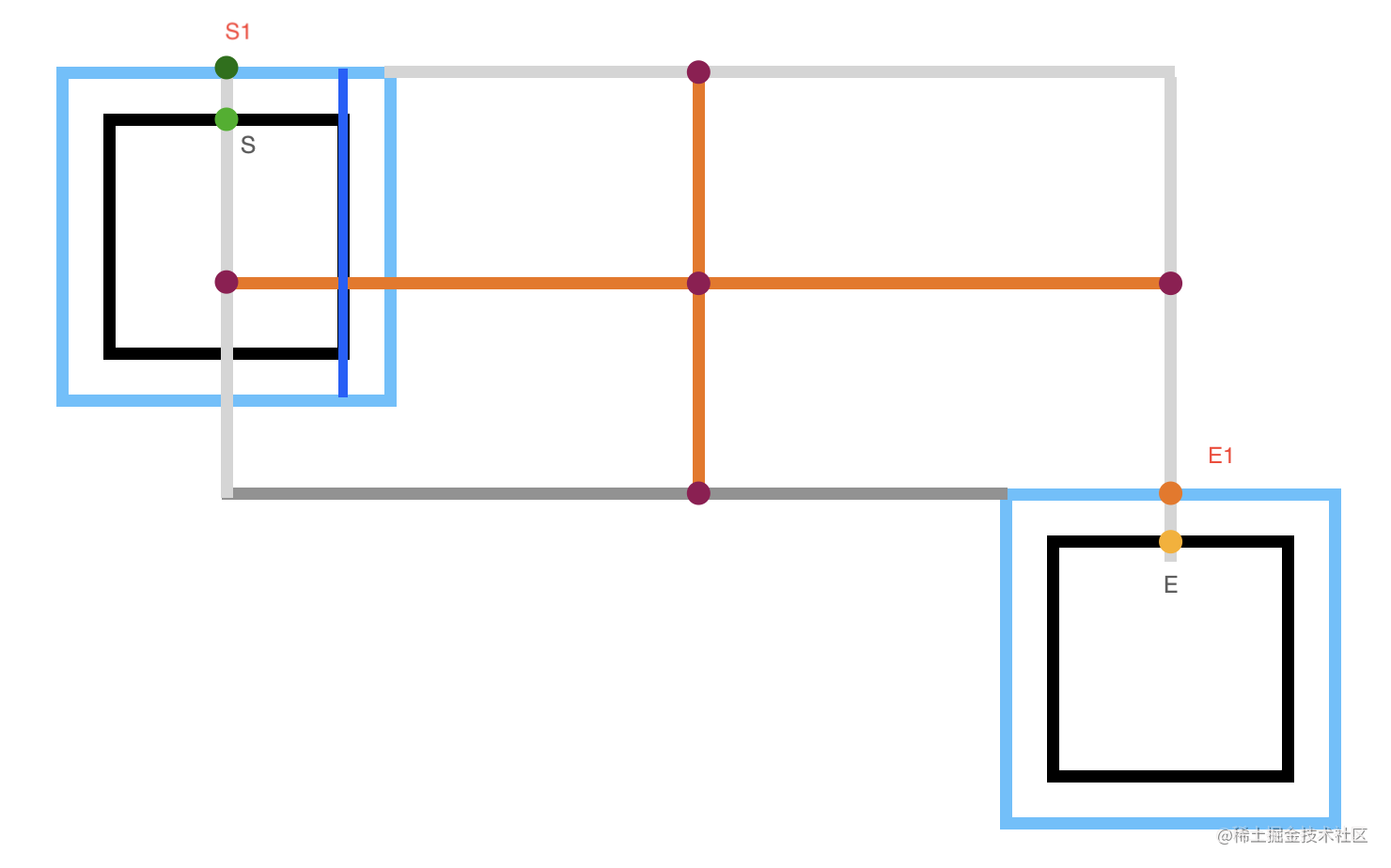``````const extendBox = (box: number[][], d: number) => {
const result = box.map((item) => [...item]);

return result;
};

#### 起点被结束盒子包含且结束点被开始盒子包含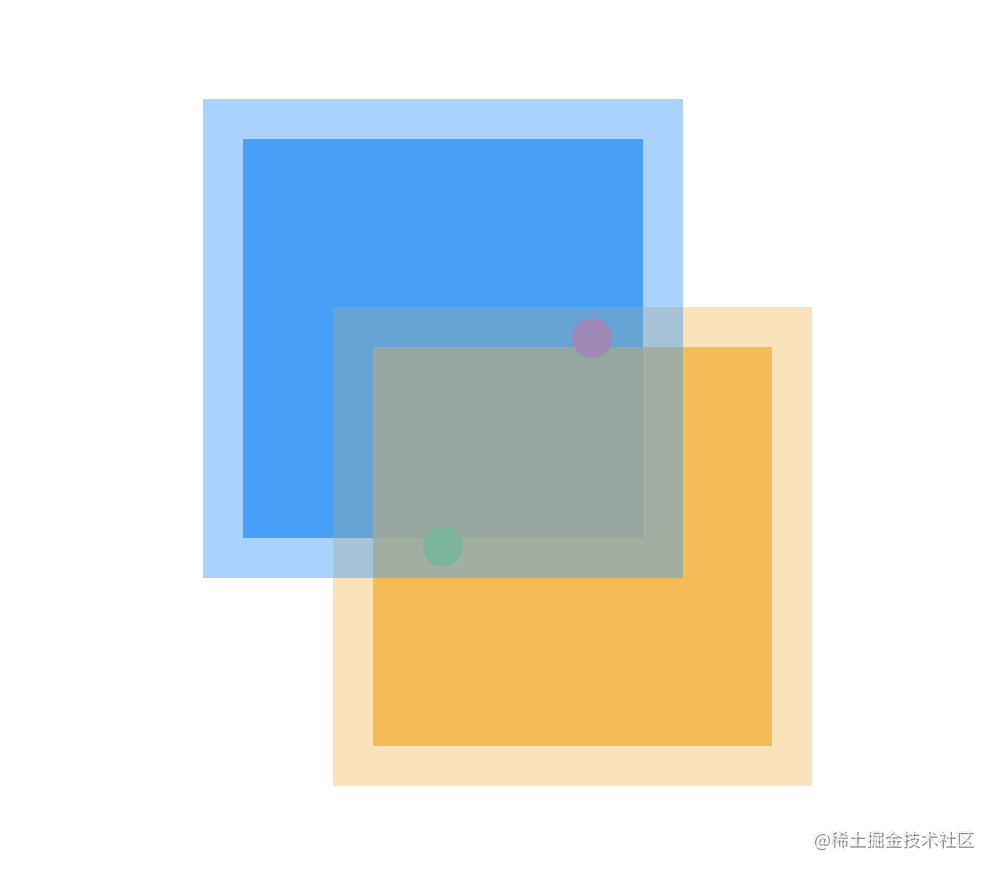#### 开始盒子和结束盒子相交（排除上面的情况）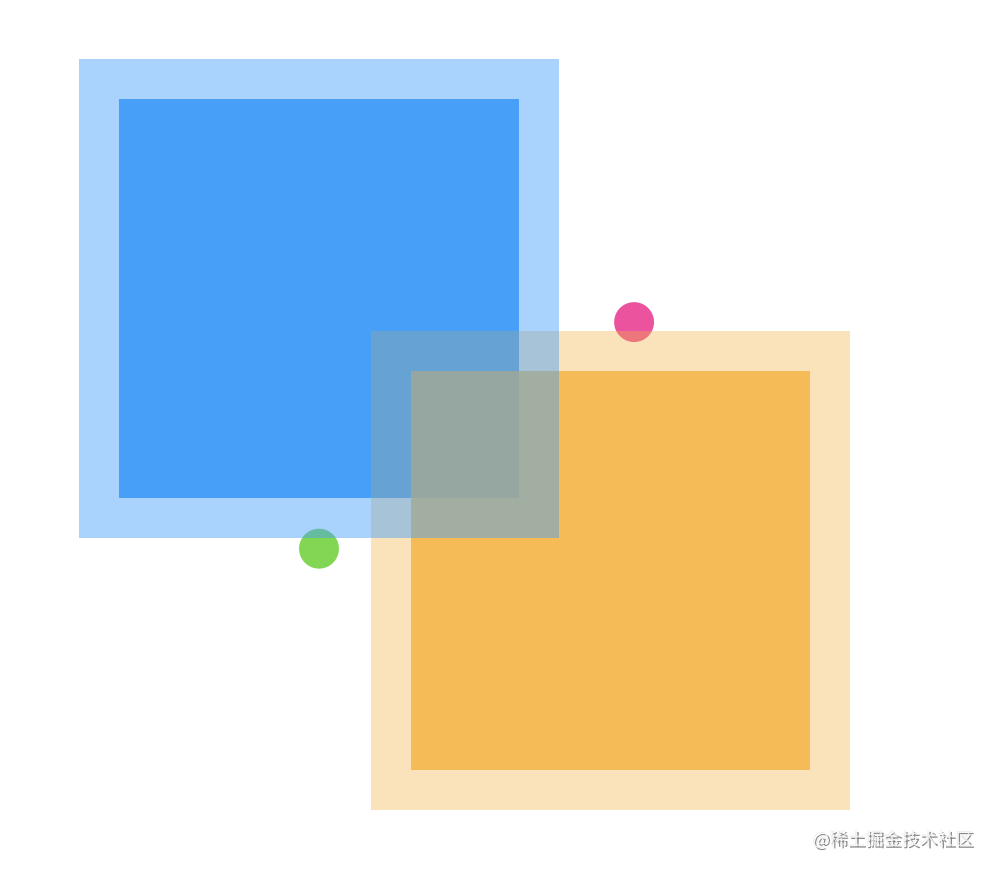### 计算约束盒子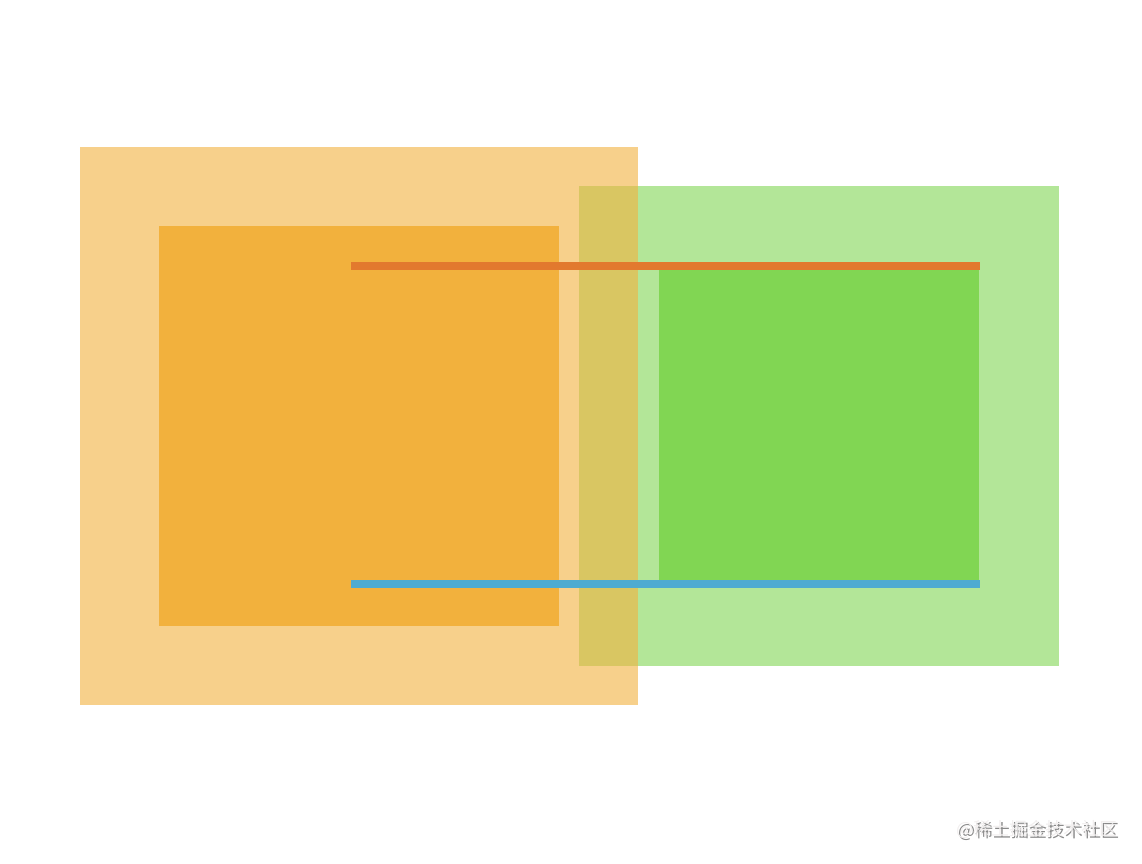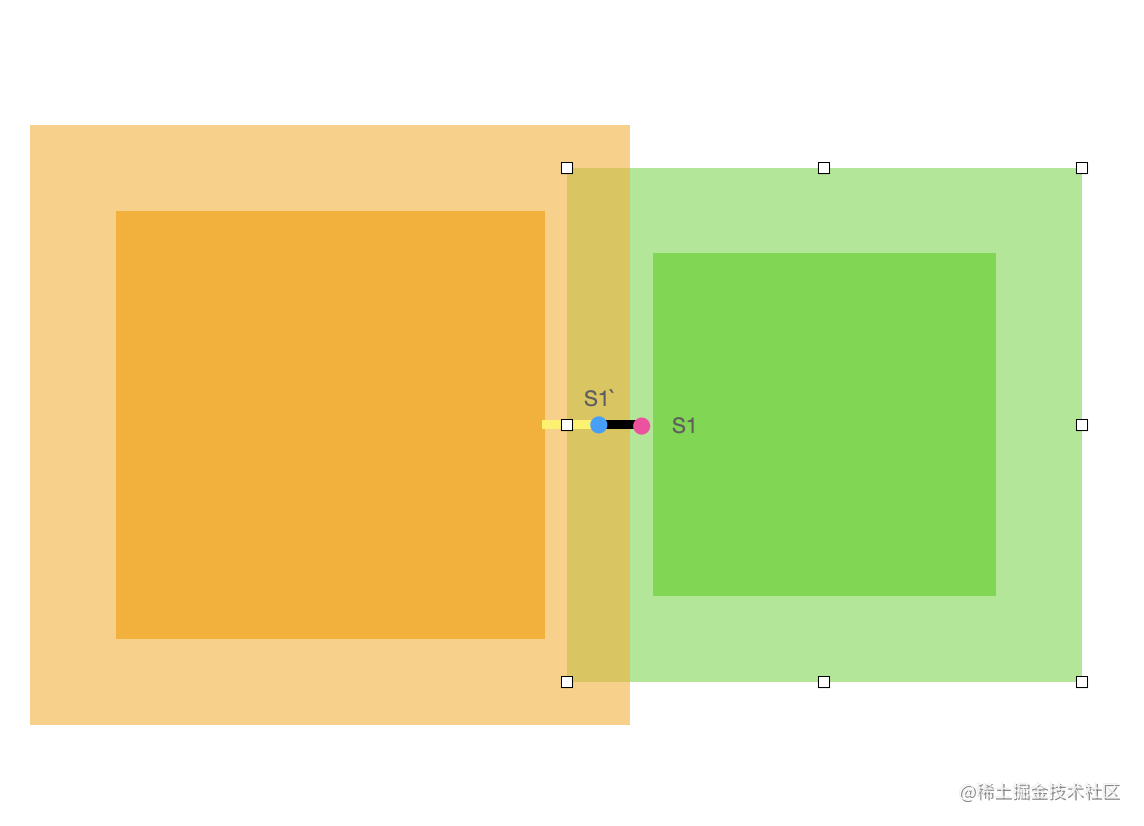`S1` 是未修正前的位置，当 `padding` 减少时，`S1` 的 x 也应该减少，为 `S1'`

Amit’s A* Pages

Heuristics